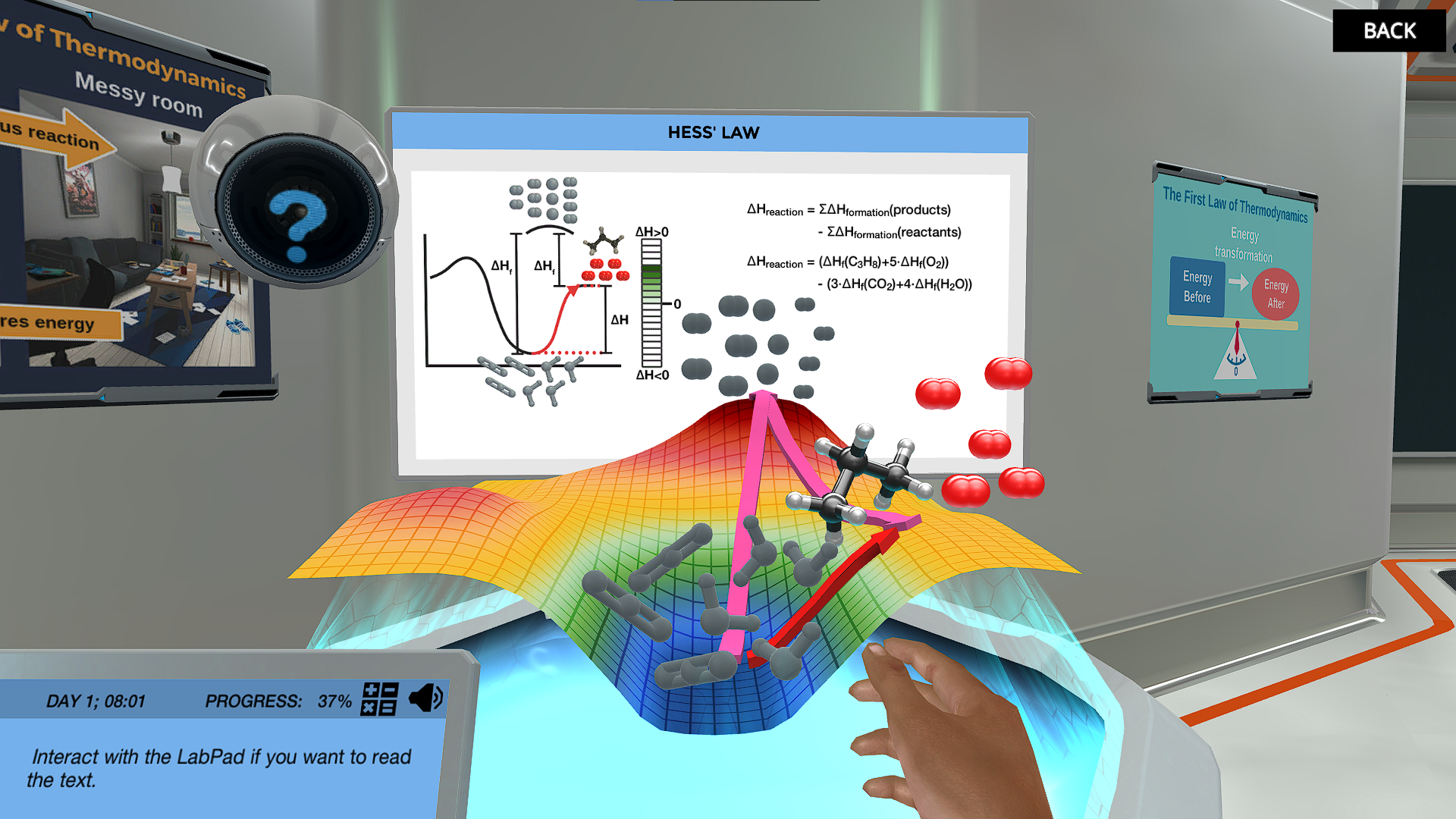# Energy Surfaces and Spontaneous Reactions Virtual Lab

Learn the core concepts of thermodynamics and discover how chemical reactions can be represented with energy surfaces. Determine which chemical reactions are spontaneous.Try our lab safety simulation

Discover one of our 200+ learning simulations today

• Description
• Features

## About the Energy Surfaces and Spontaneous Reactions Virtual Lab

This short, targeted simulation is adapted from the full-length “Basic Chemistry Thermodynamics” simulation.

Energy is one of the great subject matters of our time, but what is energy actually? In this simulation you will learn the fundamental thermodynamic concepts of enthalpy, entropy and Gibbs free energy. You will study chemical reactions and Hess’s law with an energy surface. Learn about entropy and reaction spontaneity. Can you determine which chemical reactions are spontaneous?

Exothermic and endothermic reactions

In this simulation, you can play around with the energy levels of reactants and products on a virtual energy surface to visualise the enthalpy of the reaction and learn about the difference between endothermic and exothermic reactions. Next visualise the combustion of propane on the energy surface and use Hess’s law to calculate the overall enthalpy from the enthalpies of reaction and formation.

The fundamental concepts of thermodynamics

The second law of thermodynamics and the core concepts of enthalpy, entropy and Gibbs free energy are introduced in this simulation. Discover the second law of thermodynamic and the concept of entropy then apply what you learnt to the reaction on the energy surface. Understand the relationship between enthalpy, entropy, and gibbs free energy. The concept of reaction spontaneity is introduced and linked to the concept of Gibbs free energy.

Spontaneous reactions

The temperature dependence of gibbs free energy and how this impacts reaction spontaneity is explored in an interactive game. Work out which chemical reactions are spontaneous and make a hypothesis about whether they remain spontaneous at higher temperatures. Explore the change in gibbs free energy and as the temperature changes. Will your hypothesis be correct?

Get started now

Learn the core concepts of thermodynamics and discover how chemical reactions can be represented with energy surfaces. Determine which chemical reactions are spontaneous.

Techniques in lab
Learning objectives

At the end of this simulation, you will be able to...

• Define the core thermodynamics concepts of entropy, enthalpy, and free Gibbs energy
• Explain the second law of thermodynamics
• Understand and apply the concept of reaction spontaneity
• Explain the differences between the enthalpy of combustion, and enthalpy of formation
• Present Hess's law in connection with performing enthalpy change calculations
• Present the concepts of exothermic and endothermic reactions
Simulation features

Length: 15 Minutes
Accessibility mode: Available
Languages: English (United States)

NGSS

PS3.B-H2

IB

Chemistry 15.2 Entropy and spontaneity

AP

Chemistry 9.3 Gibbs free energy and thermodynamic favorability